Next: Conclusions Up: Results Previous: Regression coefficients

## Fits to experimental data

The regression coefficients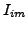obtained in the present work can be used to evaluate the possibility of readjusting the EDF coupling constants so as to better describe the experimental data. Indeed, if for a given set of coupling constants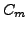the self-consistent s.p. energies are equal to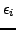, then corrections to coupling constants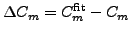are given by: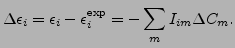(12)

The matrixbeing rectangular, it cannot be simply inverted, but, instead, one can use its singular value decomposition  to determine the result, i.e.,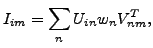(13)

where columns of matrices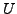and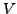are orthogonal and singular values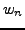are positive. In our case, the number of experimental data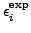is much larger than the number of coupling constants, which is equal to 12. Therefore, the possibility of describing experimental data clearly depends on whether the column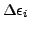can be expressed as a linear combination of 12 orthogonal columns of matrix. In any case, the result of the least-square fit to experimental data is given by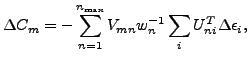(14)

for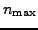=12.

Singular valuesobtained for regression coefficientscorresponding to three Skyrme functionals are shown in the lower panel of Fig. 16. Independently of which functional is concerned, the singular values decrease exponentially from about 1.3 to 0.001. Again, this illustrates a great universality of the regression coefficients.

On the one hand, as seen in Eq. (13), small singular valuescorrespond to columns of matricesandthat have very small influence on the matrix of regression coefficients. On the other hand, these same small singular values have very large impact on the coupling constants in Eq. (14). Therefore, expression (14) should be used for non-maximum values of, i.e., by cutting off the small singular values. By that, one removes from the final result those features of the regression coefficients that are poorly defined, and at the same time one keeps only those linear combinations of coupling constants that are well determined by data.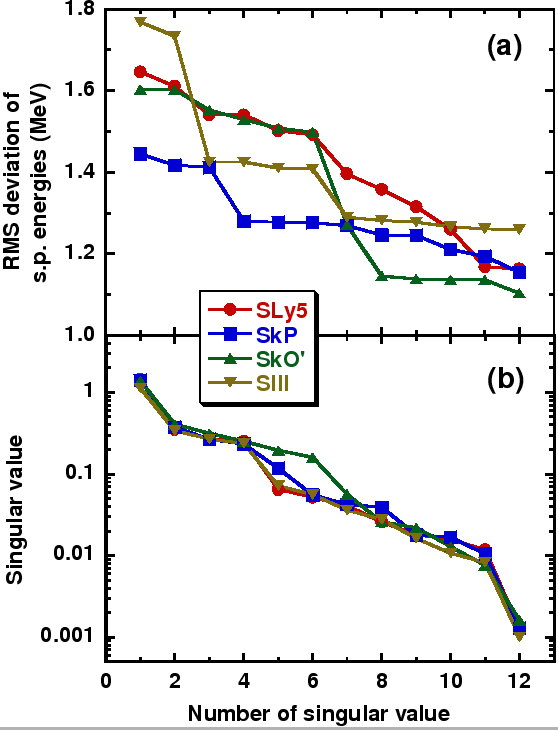In our analysis, for experimental data we adopted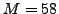proton and neutron negative s.p. listed in Ref. . Since here we study bare theoretical s.p. energies, we have also subtracted from the experimental values the calculated mass polarization corrections related to the one-body center-of-mass correction . In the upper panel of Fig. 16, we show the rms deviations,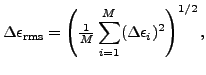(15)

of the fitted s.p. energies, obtained for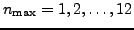. For the standard parametrizations studied here, one obtains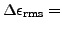1.73, 1.45, 1.61, and 1.77MeV for SLy5, SkP, SkO', and SIII functionals, respectively. At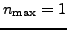, one obtains the rms deviations that are only marginally better. With increasing, the results gradually improve, and at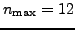(for the full least-square fit) they reach limiting values of about 1.10-1.25MeV.

At this point, we would like to stress that in this study we fit only the s.p. energies, while in reality many other observables should be (and were in the past) included in the fitting procedure. In particular, in Ref.  the s.p. energies along with global nuclear properties were used to fit the Skyrme force parameters. However, our results indicate that there exist limiting lower values of the rms deviations (15). By adding other observables to the fit, one can only obtain higher values thereof, i.e., worse descriptions of the s.p. energies.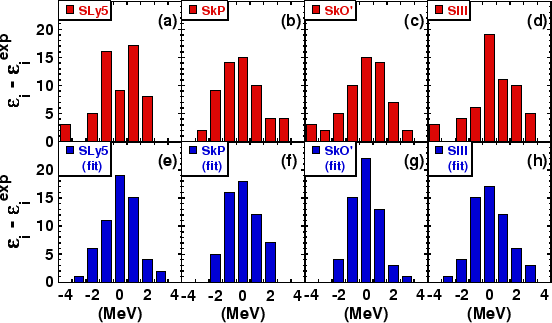Although in particular cases, some combinations of the coupling constants are especially important, i.e., at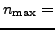7-8 for SkO' or at4 for SkP, the rms deviations decrease rather steadily (especially for SLy5), and do not show pronounced affects at low values of, observed previously for the total binding energies, see Fig. 2 in Ref. . On the one hand, this means that the experimental values of the s.p. energies may, in principle, determine values of more coupling constants than those of the total binding energies. On the other hand, the limiting value of1.1MeV, which can be obtained by refitting the standard functionals, is rather disappointing. Fig. 17 shows histograms of residuals, Eq. (12), for standard (upper panels) and linearly refitted (lower panels) Skyrme functionals. One can see again that the improvement obtained by the fit is not very spectacular, with quite a number of s.p. energies differing from experiment by 1MeV or more. This indicates, that to obtain a better agreement with experimental one may have to extend the form of the standard Skyrme functional.Next: Conclusions Up: Results Previous: Regression coefficients
Jacek Dobaczewski 2008-05-18